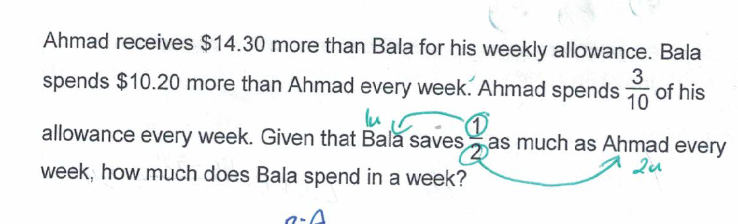# QuestionHi appreciate some help to solve the problem. thank you

Allowance                   Spent                                         Saved

Bala                  [u]                     [–(3/10)(u+14.30)–][10.20]            [ —-? —]

Saved = Allowance – Spent

Bala (Saved) = u – [(3/10)(u+14.30) + 10.20]  = u – (3/10)u – (42.9/10) – 10.20

Ahmad(Saved) = 2 x Bala (Saved)

7/10(u+14.30) = 2 { u – (3/10)u – (42.9/10) – 10.20 }     <—– x 10 on both sides,

7(u+14.30) =  20u -6u – 85.8 – 204

7u + 100.1 = 14u – 289.8

7u = 389.9

u = 55.70

Bala (Spent) = (3/10)(u+14.30) + 10.20 = (3/10)(55.70+14.30) + 10.20 = 21+10.20 = 31.20

Hence Bala spent \$31.20 in a week